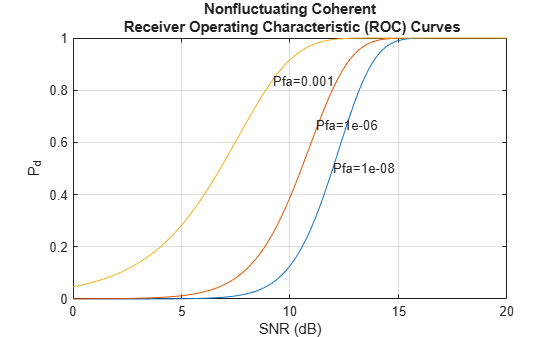# rocpfa

Receiver operating characteristic curves by false-alarm probability

## Syntax

``````[Pd,SNR] = rocpfa(Pfa)``````
``````[Pd,SNR] = rocpfa(Pfa,Name=Value)``````
``rocpfa(...)``

## Description

``````[Pd,SNR] = rocpfa(Pfa)``` returns the single-pulse detection probabilities, `Pd`, and required SNR values, `SNR`, for the false-alarm probabilities in the row or column vector `Pfa`. By default, for each false-alarm probability, the detection probabilities are computed for 101 equally spaced SNR values between 0 and 20 dB. The ROC curve is constructed assuming a single pulse in coherent receiver with a nonfluctuating target.```
``````[Pd,SNR] = rocpfa(Pfa,Name=Value)``` returns detection probabilities and SNR values with additional options specified by one or more name-value arguments.```

example

``rocpfa(...)` plots the ROC curves.`

## Examples

collapse all

Plot ROC curves for false-alarm probabilities of `1e-8`, `1e-6`, and `1e-3`, assuming no pulse integration.

```Pfa = [1e-8 1e-6 1e-3]; rocpfa(Pfa,SignalType="NonfluctuatingCoherent")```## Input Arguments

collapse all

False-alarm probabilities, specified as a row or column vector.

Example: `[1e-8 1e-6 1e-3]`

Data Types: `double`

### Name-Value Arguments

Specify optional pairs of arguments as `Name1=Value1,...,NameN=ValueN`, where `Name` is the argument name and `Value` is the corresponding value. Name-value arguments must appear after other arguments, but the order of the pairs does not matter.

Example: `MaxSNR=15,NumPoints=64,NumPulses=10`

Before R2021a, use commas to separate each name and value, and enclose `Name` in quotes.

Example: `'MaxSNR',15,'NumPoints',64,'NumPulses',10`

Maximum SNR to include in the ROC calculation, specified as a positive scalar.

Data Types: `double`

Minimum SNR to include in the ROC calculation, specified as a positive scalar.

Data Types: `double`

Number of SNR values to use when calculating the ROC curves, specified as a positive integer. The actual values are equally spaced between `MinSNR` and `MaxSNR`.

Data Types: `double`

Number of pulses to integrate when calculating the ROC curves, specified as a positive integer. A value of `1` indicates no pulse integration.

Data Types: `double`

This property specifies the type of received signal or, equivalently, the probability density functions (PDF) used to compute the ROC. Valid values are: `"Real"`, `"NonfluctuatingCoherent"`, `"NonfluctuatingNoncoherent"`, `"Swerling1"`, `"Swerling2"`, `"Swerling3"`, and `"Swerling4"`. Values are not case sensitive.

The `"NonfluctuatingCoherent"` signal type assumes that the noise in the received signal is a complex-valued, Gaussian random variable. This variable has independent zero-mean real and imaginary parts each with variance σ2/2 under the null hypothesis. In the case of a single pulse in a coherent receiver with complex white Gaussian noise, the probability of detection, PD, for a given false-alarm probability, PFA is:

`${P}_{D}=\frac{1}{2}\text{erfc}\left({\text{erfc}}^{-1}\left(2{P}_{FA}\right)-\sqrt{\chi }\right)$`

where `erfc` and `erfc-1` are the complementary error function and that function’s inverse, and χ is the SNR not expressed in decibels.

For details about the other supported signal types, see .

Data Types: `char` | `string`

## Output Arguments

collapse all

Detection probabilities corresponding to the false-alarm probabilities, returned as a vector. For each false-alarm probability in `Pfa`, `Pd` contains one column of detection probabilities.

Signal-to-noise ratios, returned as a column vector. By default, the SNR values are 101 equally spaced values between 0 and 20. To change the range of SNR values, use the optional `MinSNR` or `MaxSNR` input argument. To change the number of SNR values, use the optional `NumPoints` input argument.

## Version History

Introduced in R2011a